• Use pandas to load the latest updated data from Yahoo Finance
• Explore and understand the dataset
• Viewing the data
• Correlation Analysis
• Graphing using matplotlib
• Predict the return of the stocks in the next period - prediction analysis
• Simple Linear Analysis
• K Nearest Neighbor (KNN)

In this demonstration, we will use pandas webreader. This is an extension of pandas library to communicate with most updated financial data.

In :
import pandas as pd
import datetime
from pandas import Series, DataFrame

start = datetime.datetime(2019, 1, 1)
end = datetime.datetime(2020, 3, 1)

df = web.DataReader("AAPL", 'yahoo', start, end)
df.tail()

Out:
High Low Open Close Volume Adj Close
Date
2020-02-24 304.179993 289.230011 297.260010 298.179993 55548800.0 298.179993
2020-02-25 302.529999 286.130005 300.950012 288.079987 57668400.0 288.079987
2020-02-26 297.880005 286.500000 286.529999 292.649994 49513700.0 292.649994
2020-02-27 286.000000 272.959991 281.100006 273.519989 80151400.0 273.519989
2020-02-28 278.410004 256.369995 257.260010 273.359985 106721200.0 273.359985

Get the dimension of dataframe

In :
df.shape

Out:
(292, 6)

## Exploring the data¶

First we will need to explore the data. This means understanding the stocks data trend thoroughly by finding key measures

• rolling mean (moving average) -- to determine trend
• return deviation -- to determine return deviation
In :
close_px = df['Adj Close']
mavg = close_px.rolling(window=100).mean()
mavg.tail(10)

Out:
Date
2020-02-14    272.504052
2020-02-18    273.528906
2020-02-19    274.566640
2020-02-20    275.582512
2020-02-21    276.536527
2020-02-24    277.290618
2020-02-25    277.937542
2020-02-26    278.686165
2020-02-27    279.224987
2020-02-28    279.700641
Name: Adj Close, dtype: float64
In :
%matplotlib inline
import matplotlib.pyplot as plt
from matplotlib import style

# Adjusting the size of matplotlib
import matplotlib as mpl
mpl.rc('figure', figsize=(8, 7))
mpl.__version__

# Adjusting the style of matplotlib
style.use('ggplot')

close_px.plot(label='AAPL')
mavg.plot(label='mavg')
plt.legend()

Out:
<matplotlib.legend.Legend at 0x1a1e2cf050>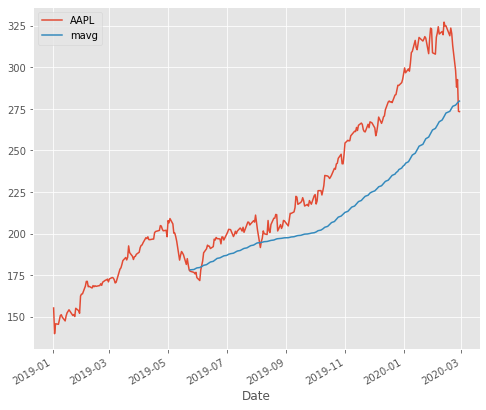Returns defined as:  $$r_t = \frac{p_t - p_{t-1}}{p_{t-1}} = \frac{p_t}{p_{t-1}} - 1$$

In :
rets = close_px / close_px.shift(1) - 1

Out:
Date
2019-01-02         NaN
2019-01-03   -0.099607
2019-01-04    0.042689
2019-01-07   -0.002226
2019-01-08    0.019063
Name: Adj Close, dtype: float64
In :
rets.plot(label='return')

Out:
<matplotlib.axes._subplots.AxesSubplot at 0x1a1e837b50>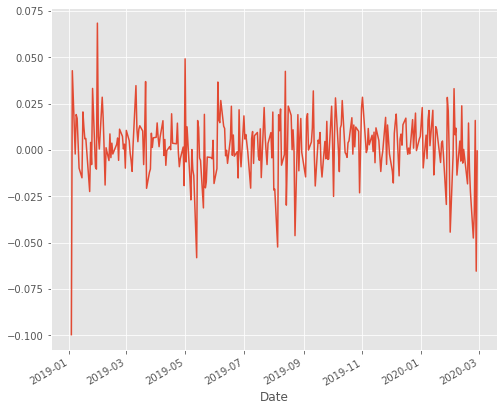## Peer group analysis & correlation¶

1. How does one company perform in relation to its competitors
2. What are the correlations among these companies
In :
dfcomp = web.DataReader(['AAPL', 'GE', 'GOOG', 'IBM', 'MSFT'],'yahoo',
start=start,
dfcomp.tail()

Out:
Symbols AAPL GE GOOG IBM MSFT
Date
2020-02-24 298.179993 11.848234 1421.589966 146.429993 170.889999
2020-02-25 288.079987 11.308769 1388.449951 141.710007 168.070007
2020-02-26 292.649994 10.939137 1393.180054 139.750000 170.169998
2020-02-27 273.519989 10.379693 1318.089966 133.110001 158.179993
2020-02-28 273.359985 10.869206 1339.329956 130.149994 162.009995

Get the dimension of dataframes

In :
dfcomp.shape

Out:
(292, 5)
In :
retscomp = dfcomp.pct_change()

corr = retscomp.corr()
corr

Out:
Symbols AAPL GE GOOG IBM MSFT
Symbols
AAPL 1.000000 0.368629 0.581641 0.434626 0.661574
GE 0.368629 1.000000 0.263895 0.313710 0.311480
GOOG 0.581641 0.263895 1.000000 0.390734 0.649028
IBM 0.434626 0.313710 0.390734 1.000000 0.488553
MSFT 0.661574 0.311480 0.649028 0.488553 1.000000
In :
plt.scatter(retscomp.AAPL, retscomp.GE)
plt.xlabel('Returns AAPL')
plt.ylabel('Returns GE')

Out:
Text(0, 0.5, 'Returns GE')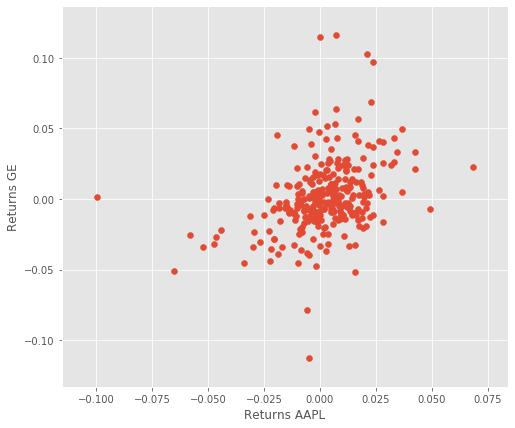You can do scatter matrix with all the competitors data and find the kde of each m KDE will determine if your chart is more normally distributed leaning to the

• left: Returns are more likely to be negative in the long run
• centre: Returns are more likely to be 0 in the long run
• right: Returns are more likely to be positive in the long run
In :
from pandas.plotting import scatter_matrix
pd.plotting.scatter_matrix(retscomp, diagonal='kde', figsize=(10, 10));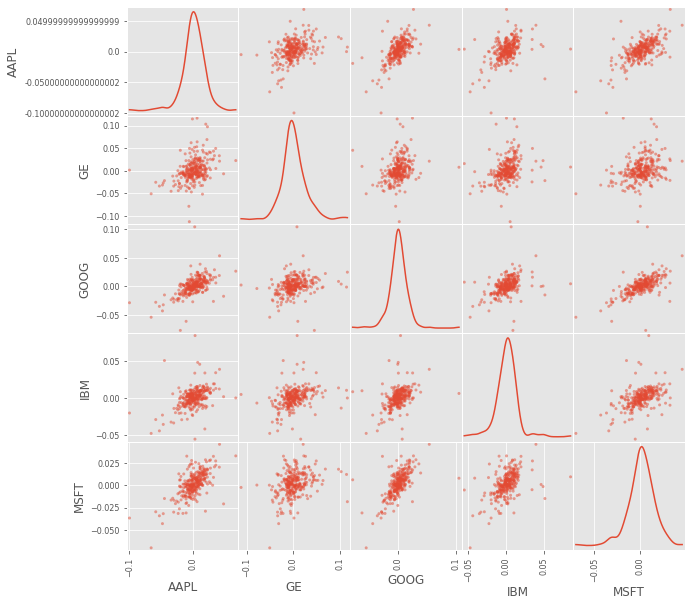In :
plt.imshow(corr, cmap='hot', interpolation='none')
plt.colorbar()
plt.xticks(range(len(corr)), corr.columns)
plt.yticks(range(len(corr)), corr.columns);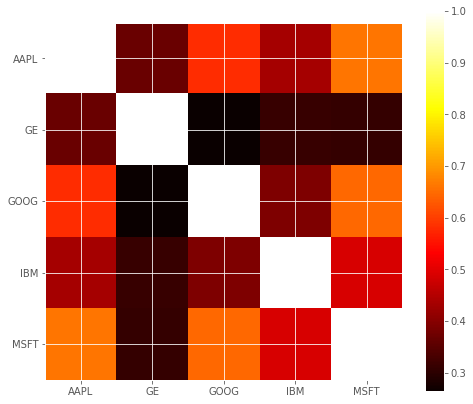## Stocks mean and risk calculation¶

Which stocks are risky and bearing returns

First, looking at kurtosis and skewness

In :
plt.scatter(retscomp.mean(), retscomp.std())
plt.xlabel('Expected returns')
plt.ylabel('Risk')
for label, x, y in zip(retscomp.columns, retscomp.mean(), retscomp.std()):
plt.annotate(
label,
xy = (x, y), xytext = (20, -20),
textcoords = 'offset points', ha = 'right', va = 'bottom',
bbox = dict(boxstyle = 'round,pad=0.5', fc = 'yellow', alpha = 0.5),
arrowprops = dict(arrowstyle = '->', connectionstyle = 'arc3,rad=0'))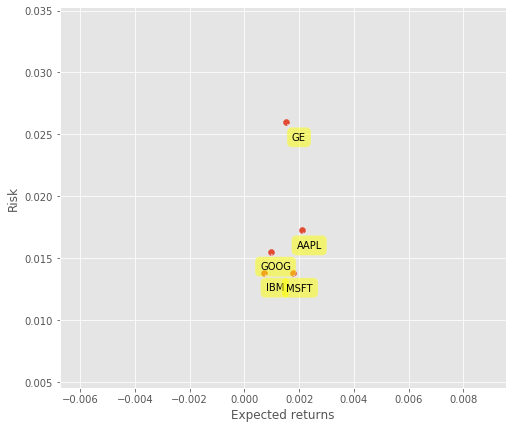# Predicting the stock's price¶

To predict the return of the stocks in the next period - Prediction analysis

• Simple Linear Analysis
• K Nearest Neighbor (KNN)

But first, let us extract the necessary columns first from df:

• HL Percentage
• PCT Change
In :
df.head()

Out:
High Low Open Close Volume Adj Close
Date
2019-01-02 158.850006 154.229996 154.889999 157.919998 37039700.0 155.214005
2019-01-03 145.720001 142.000000 143.979996 142.190002 91312200.0 139.753540
2019-01-04 148.550003 143.800003 144.529999 148.259995 58607100.0 145.719513
2019-01-07 148.830002 145.899994 148.699997 147.929993 54777800.0 145.395203
2019-01-08 151.820007 148.520004 149.559998 150.750000 41025300.0 148.166870
In :
dfreg = df.loc[:,['Adj Close','Volume']]
dfreg['HL_PCT'] = (df['High'] - df['Low']) / df['Close'] * 100.0
dfreg['PCT_change'] = (df['Close'] - df['Open']) / df['Open'] * 100.0

Out:
Date
2019-01-02 155.214005 37039700.0 2.925539 1.956226
2019-01-03 139.753540 91312200.0 2.616219 -1.243224
2019-01-04 145.719513 58607100.0 3.203831 2.580776
2019-01-07 145.395203 54777800.0 1.980672 -0.517824
2019-01-08 148.166870 41025300.0 2.189057 0.795669
In :
import math
import numpy as np
from sklearn import preprocessing, svm
from sklearn.model_selection import cross_validate

# Drop missing value
dfreg.fillna(value=-99999, inplace=True)

print(dfreg.shape)
# We want to separate 1 percent of the data to forecast
forecast_out = int(math.ceil(0.01 * len(dfreg)))

# Separating the label here, we want to predict the AdjClose
dfreg['label'] = dfreg[forecast_col].shift(-forecast_out)
X = np.array(dfreg.drop(['label'], 1))

# Scale the X so that everyone can have the same distribution for linear regression
X = preprocessing.scale(X)

# Finally We want to find Data Series of late X and early X (train) for model generation and evaluation
X_lately = X[-forecast_out:]
X = X[:-forecast_out]

# Separate label and identify it as y
y = np.array(dfreg['label'])
y = y[:-forecast_out]

print('Dimension of X',X.shape)
print('Dimension of y',y.shape)

(292, 4)
Dimension of X (289, 4)
Dimension of y (289,)

In :
# Separation of training and testing of model by cross validation train test split
from sklearn import metrics
import sklearn.model_selection as model_selection
X_train, X_test, y_train, y_test = model_selection.train_test_split(X, y, train_size=0.75,test_size=0.25)


# Model Generation¶

• To predict the return of the stocks in the next period - Prediction analysis
• Simple Linear Analysis
• K Nearest Neighbor (KNN)

## Building the model¶

In :
from sklearn.linear_model import LinearRegression
from sklearn.neighbors import KNeighborsRegressor

from sklearn.linear_model import Ridge
from sklearn.preprocessing import PolynomialFeatures
from sklearn.pipeline import make_pipeline

# Linear regression
clfreg = LinearRegression(n_jobs=-1)
clfreg.fit(X_train, y_train)

clfpoly2 = make_pipeline(PolynomialFeatures(2), Ridge())
clfpoly2.fit(X_train, y_train)

clfpoly3 = make_pipeline(PolynomialFeatures(3), Ridge())
clfpoly3.fit(X_train, y_train)

# KNN Regression
clfknn = KNeighborsRegressor(n_neighbors=2)
clfknn.fit(X_train, y_train)

Out:
KNeighborsRegressor(algorithm='auto', leaf_size=30, metric='minkowski',
metric_params=None, n_jobs=None, n_neighbors=2, p=2,
weights='uniform')

## Test the model¶

In :
confidencereg = clfreg.score(X_test, y_test)
confidencepoly2 = clfpoly2.score(X_test,y_test)
confidencepoly3 = clfpoly3.score(X_test,y_test)
confidenceknn = clfknn.score(X_test, y_test)

print("The linear regression confidence is ",confidencereg)
print("The quadratic regression 2 confidence is ",confidencepoly2)
print("The quadratic regression 3 confidence is ",confidencepoly3)
print("The knn regression confidence is ",confidenceknn)

The linear regression confidence is  0.9762673467427405
The quadratic regression 2 confidence is  0.9791518854177363
The quadratic regression 3 confidence is  0.8340197863651472
The knn regression confidence is  0.8516089175379271


Quadratic regression has marginally better performance.

However, for the purpose of this post, and keeping to the basics -- we're going to focus on linear regression.

Bute note, there are many issues to consider, especially with different companies that have different price trajectories over time.

We need to plot out the graph to make the most sense of it.

In :
# Printing the forecast
forecast_set = clfreg.predict(X_lately)
dfreg['Forecast'] = np.nan
print(forecast_set, confidencereg, forecast_out)

[291.41418218 277.70044098 267.91862041] 0.9762673467427405 3


# Plotting the Prediction¶

In :
last_date = dfreg.iloc[-1].name
last_unix = last_date
next_unix = last_unix + datetime.timedelta(days=5)

for i in forecast_set:
next_date = next_unix
next_unix += datetime.timedelta(days=5)
dfreg.loc[next_date] = [np.nan for _ in range(len(dfreg.columns)-1)]+[i]

In :
dfreg['Adj Close'].tail(1000).plot()
dfreg['Forecast'].tail(1000).plot()
plt.legend(loc=4)
plt.xlabel('Date')
plt.ylabel('Price')
plt.show()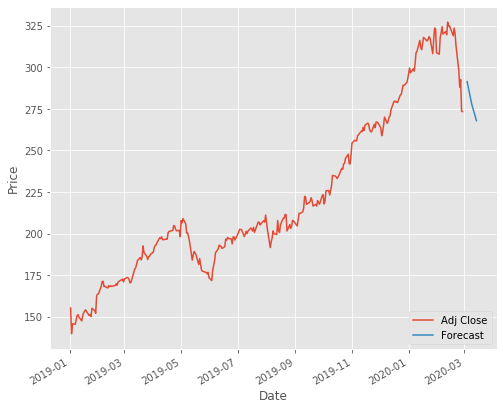In [ ]:


In [ ]: